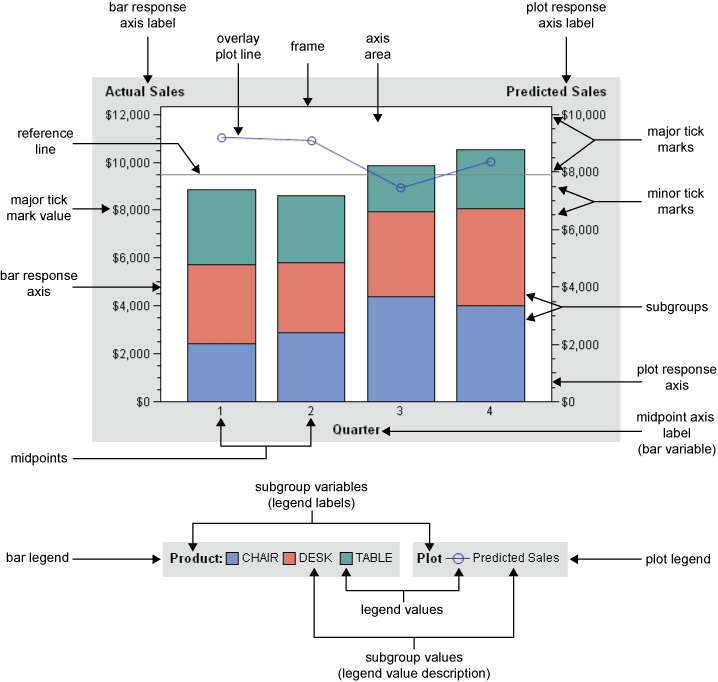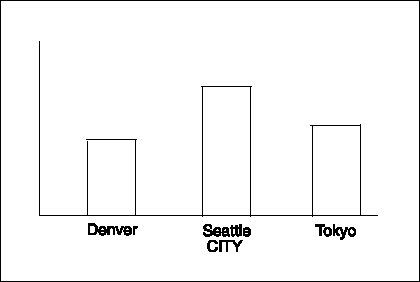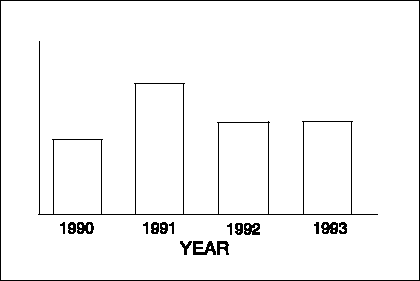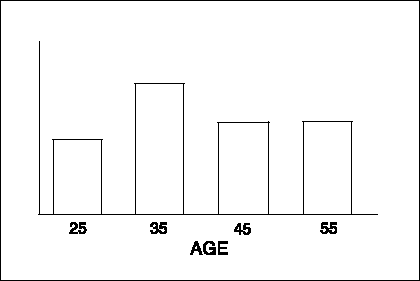The GBARLINE Procedure

# Concepts

The GBARLINE procedure produces a bar chart based on the values of a chart variable and an optional response variable (SUMVAR= option). The computed statistic can be set with the TYPE= option. Each line chart uses the same chart variable and has an optional response variable (SUMVAR= option). A computed statistic can be set with the TYPE= option.

Parts of a Bar-Line Chart illustrates the parts of a bar-line chart.Bar-line charts have three axes:

• a midpoint axis that shows the categories of data, based on the chart variable

• a left response axis that displays the scale of values for the bar statistic (based on the response variable, if specified)

• a right response axis that displays the scale of values for the line statistic (based on the response variable, if specified)

The response axes are divided into evenly spaced intervals identified with major tick marks that are labeled with the corresponding statistic value. Minor tick marks are evenly distributed between the major tick marks. Each axis is labeled with the variable name or label. The right response axis is scaled to accommodate all the line variable response values when multiple PLOT statements are present.

The chart variable is the variable in the input data set whose value determines the categories of data represented by the bar and lines. The chart variable generates the midpoints to which each observation in the data set contribute.

A character chart variable is always discrete.

Midpoints are the values of the chart variable that identify categories of data. By default, midpoints are selected or calculated by the procedure. The way the procedure handles the midpoints depends on whether the values of the chart variable are character, discrete numeric, or continuous numeric.

### Character Values

A character chart variable generates a midpoint for each unique value of the variable. In the following example, the chart variable CITY contains the names of three different cities, and each city is a midpoint, resulting in three midpoints for the chart:By default, character midpoints are arranged in alphabetic order. If a character variable has an associated format, then the values are arranged in order of the formatted values.

### Discrete Numeric Values

A numeric chart variable used with the DISCRETE option generates a midpoint for each unique value of the chart variable. In the following example, the numeric variable YEAR used with the DISCRETE option produces one midpoint for each year:

Discrete Numeric MidpointsBy default, numeric midpoints are arranged in ascending order of the chart variable. If the numeric variable has an associated format, then each formatted value generates a separate midpoint. Formatted numeric variables are arranged in ascending order according to their unformatted numeric values.

### Continuous Numeric Values

A continuous numeric variable generates midpoints that represent ranges of values. By default, the GBARLINE procedure determines the number of uniform ranges (LEVELS), calculates the number of observations in each range, and then computes the TYPE= statistic based on this frequency. A value that falls exactly on a range boundary is placed in the higher range.

In the following example, the numeric variable AGE has been divided into five equal levels that span the data range. The horizontal axis tick values are at the midpoint of each level.

Continuous Numeric MidpointsBy default, midpoints of ranges are arranged in ascending order.

### Selecting and Ordering Midpoints

For character or discrete numeric values, you can use the MIDPOINTS= option to rearrange the midpoints or to exclude midpoints from the chart. For example, to change the default alphabetic order of the midpoints in Character Midpoints, specify the following midpoints:

`midpoints="Tokyo" "Denver" "Seattle"`

To exclude the midpoint for Denver, specify the following midpoints:

`midpoints="Tokyo" "Seattle"`

In this case, values excluded by the option are not included in the calculation of the chart statistic.

You can order or select discrete numeric midpoint values just as you do character values, but you omit the quotation marks when specifying numeric values.

For continuous numeric variables, use the LEVELS= or MIDPOINTS= option to change the number of midpoints, to control the range of values each midpoint represents, or to change the order of the midpoints. To control the range of values each midpoint represents, use the MIDPOINTS= option to specify the midpoint value of each range. For example, to select the ranges 20-29, 30-39, and 40-49, specify the following values:

`midpoints=25 35 45;`

Alternatively, to select the number of midpoints that you want and let the procedure calculate the ranges and midpoints, use the LEVELS= option.

You can also use formats to control the ranges of continuous numeric variables, but in that case the values are no longer continuous but become discrete.

Note:   You cannot use the MIDPOINTS= option to exclude continuous numeric values from the chart because values below or above the ranges specified by the option are automatically included in the first and last midpoints. To exclude continuous numeric values from a chart, use a WHERE statement in a DATA step or the WHERE= data set option.Response variables can be specified for either the bar chart or any line plot with the SUMVAR= option.

For example:

`  BAR age  / DISCRETE SUMVAR=weight;  PLOT / SUMVAR=height;`

When you specify a response variable, the only statistics available are SUM or MEAN, with SUM being the default. To change the statistic, you specify the TYPE= option. For example, TYPE=MEAN.

If you do not specify a response variable, a summary statistic for the chart variable is computed. By default it is FREQ (frequency). You can use the TYPE= option to indicate another statistic: PERCENT, CFREQ (cumulative frequency) or CPERCENT (cumulative percent).

The chart statistics are the statistical values calculated for the chart variable or the response variable. When there is no response variable, the GBARLINE procedure calculates one of four possible statistics with the default being FREQ. When there is a response variable one of two possible statistics is computed with the default being SUM. You can specify the chart statistic with the TYPE= option for both the bar chart and any line plot. For the bar chart, the default statistic is frequency. For the plot variable, the default statistic is sum.

The examples given in the descriptions of these statistics assume a data set with two variables, CITY and SALES. The values of CITY are Denver, Seattle, and Tokyo. There are 21 observations: seven for Denver, nine for Seattle, and five for Tokyo.

### Frequency

The frequency statistic is the total number of observations in the data set for each midpoint. For example, seven observations of the bar variable, CITY, contain the value Denver, so the frequency for the Denver midpoint is 7.

### Cumulative Frequency

The cumulative frequency statistic adds the frequency for the current midpoint to the frequency of all of the preceding midpoints. For example, the frequency for the Denver midpoint is 7, and the frequency for the next midpoint, Seattle, is 9. Therefore, the cumulative frequency for Seattle is 16 and the cumulative frequency for Tokyo is 21.

### Percentage

The percentage statistic is calculated by dividing the frequency for each midpoint by the total frequency count for all midpoints in the chart or group and multiplying it by 100. For example, the frequency count for the Denver midpoint is 7 and the total frequency count for the chart is 21, so the percentage statistic for Denver is 33.3%.

### Cumulative Percentage

The cumulative percentage statistic adds the percentage for the current midpoint to the percentage for all of the preceding midpoints in the chart or group. For example, the percentage for the Denver midpoint is 33.3, and the percentage for the next midpoint, Seattle, is 42.9, so the cumulative percentage for Seattle is 76.2.

### Sum

The sum statistic is the total of the values, for each midpoint, for the variable specified by the SUMVAR= option. For example, if you specify SUMVAR=SALES and the values of the SALES variable for the seven Denver observations are 8734, 982, 1504, 3207, 4502, 624, and 918, the sum statistic for the Denver midpoint is 20,471.

You must use the SUMVAR= option to specify the variable for which you want the sum statistic.

### Mean

The mean statistic is the average of the values, for each midpoint, for the variable specified by the SUMVAR= option. For example, if TYPE=MEAN and SUMVAR=SALES, the mean statistic for the Denver midpoint is 2924.42.

You must use the SUMVAR= option to specify the variable for which you want the mean statistic.

### Calculating Weighted Statistics

By default, each observation is counted only once in the calculation of a chart statistic. To calculate weighted statistics in which an observation can be counted more than once, use the FREQ= option. This option identifies a variable whose values are used as a multiplier for the observation in the calculation of the statistic. If the value of the FREQ= variable is missing, zero, or negative, then the observation is excluded from the calculation.

If you use the SUMVAR= option, then the SUMVAR= variable value for an observation is multiplied by the FREQ= variable value for the observation. The product of this calculation determines the chart statistic.

For example, to use a variable called COUNT to produce weighted statistics, assign FREQ=COUNT. If you also assign the variable HEIGHT to the SUMVAR= option, then the following table shows how the values of COUNT and HEIGHT would affect the statistic calculation:

Value of COUNT Value of HEIGHT Number of times the observation is used Value used for HEIGHT
1 55 1 55
5 65 5 325
. 63 0 -
-3 60 0 -

By default, the percentage and cumulative percentage statistics are calculated based on the frequency. If you want to graph a percentage or cumulative percentage based on a sum, then you can use the FREQ= option to specify a variable to use for the sum calculation and then specify PCT as the statistic, as shown in this example:

`freq=count type=pct;`

Because the variable that is specified by the FREQ= option determines the number of times an observation is counted, the value of COUNT is the equivalent of the sum statistic.

Note:   The FREQ= option is not supported by ActiveX or Java.By default, the GBARLINE procedure ignores missing midpoint values for the chart variable. If you specify the MISSING option, then missing values are treated as a valid midpoint and are included on the axis. Missing values for the subgroup variables are always treated as valid subgroups.

When the value of the variable that is specified in the FREQ= option is missing, zero, or negative, the observation is excluded from the calculation of the chart statistic.

When the value of the variable specified in the SUMVAR= option is missing, the observation is excluded from the calculation of the chart statistic.

If all of the values for a response variable are missing for the bar chart, a midpoint is drawn, but no bar appears above it. For a line plot, no marker is drawn and the line connects the adjacent markers.

Exclude data values from a plot overlay by restricting the range of axis values with the RAXIS= options or with the ORDER= option in an AXIS statement. When an observation contains a value outside of the specified axis range, the GBARLINE procedure excludes the observation from the plot and issues a message to the log.

If you specify interpolation with a SYMBOL definition, then the values outside the axis range are excluded from interpolation calculations by default, and, as a result, can change interpolated values for the plot overlay.

To specify that values outside of the axis range are included in the interpolation calculations, use the MODE= option in a SYMBOL statement. When MODE=INCLUDE, values that fall outside of the axis range are included in interpolation calculations but excluded from the plot. The default (MODE=EXCLUDE) omits observations that are outside of the axis range from interpolation calculations. See the SYMBOL Statement for details.

Default patterns, colors, outlines, and, in some cases, images, are defined by the current style, whether that style is the default GSTYLE or one you specify with the ODS statement. You can turn off styles by specifying the NOGSTYLE system option, or you can override individual aspects of a graph's appearance by specifying PATTERN statements, SYMBOL statements, graphics options, and procedure options.

The following sections summarize pattern behavior for the GBARLINE procedure. For more information, see the PATTERN Statement and the SYMBOL Statement.

### Default Patterns, Symbols, Lines, Colors, and Outlines

The default pattern that the GBARLINE procedure uses is a solid fill. The default colors are determined by the current style and the device.

Because the system option--GSTYLE--is in effect by default, the procedure uses the style's default bar fill colors, plot line colors, widths, symbols, patterns, and outline colors when producing output. Specifically, the GBARLINE procedure uses the default values when you do not specify any of the following:

• any PATTERN statements

• the CPATTERNS= graphics options

• the COLORS= graphics options

• the COUTLINE= option in the BAR statement

• any SYMBOLS statements.

If you do not specify any of these statements or options, then the GBARLINE procedure performs the following operations:

• selects the first default fill pattern, which is always solid, and rotates it through the list of colors available in the current style, generating one solid pattern for each color. When the solid patterns are exhausted, the procedure selects the next default subgroup bar pattern (empty) and rotates it through the appropriate set of colors. It continues in this fashion until all of the required patterns have been assigned.

If you use the default style colors and the first color in the list is either black or white, the procedure does not create a pattern in that color. If you specify a color list with the COLORS= graphics option, then the procedure uses all the colors in the list to generate the patterns.

• uses the style's outline color to outline every patterned area.

• uses the style's default symbol for the initial PLOT statement points, the second default symbol for the next PLOT statement, the third default symbol for the next PLOT statement, and so on, continuing through the set of symbols belonging to that style until all the PLOT statements have been satisfied.

• connects all the plot symbols with a solid line.

If you specify the NOGSTYLE system option, the fill pattern is solid and the color comes from the device's color list. The GBARLINE procedure uses a solid fill for the bars that it rotates once through the device's default color list, skipping the foreground color. (Typically, the foreground color is the first color in the device's color list.) If no SYMBOL or PATTERN statements are in effect and the COLORS= option is not used in the GOPTIONS statement, then the plot line colors begin with the next color from the same color list used to color the bars. By doing this, the procedure prevents the plot line from being the same color as a bar fill. Specifically, GBARLINE performs the following operations:

• selects the first default fill, which is always solid, and rotates it through the color list, generating one solid pattern for each color. If the first color in the device's color list is black (or white), the procedure skips that color and begins generating patterns with the next color.

• uses the foreground color to outline every patterned area.

• selects the next default pattern fill (if it needs additional patterns), and rotates that pattern through the color list, skipping the foreground color as before. The procedure continues in this fashion until it has generated enough patterns for the chart.

• uses the device's default color to outline every patterned area.

• selects the next color in the list after the last bar color and uses it to draw the first PLOT statement symbol and connecting line.

• rotates through the color list for any subsequent PLOT statements.

If the procedure needs additional patterns, PROC GBARLINE selects the next default pattern fill (empty) and rotates it through the color list, skipping the foreground color as before. The procedure continues in this fashion until it has generated enough patterns for the chart.

Changing any of the following conditions might change or override the default behavior:

• If you specify a color list with the COLORS= option in a GOPTIONS statement and the list contains more than one color, then the procedure rotates the default solid pattern through that list, using every color, even if the foreground color is black (or white). The default outline color remains the foreground color or the color specified by the current style.

For a description of these graphics options, see Graphics Options and Device Parameters Dictionary.

### User-Defined Patterns, Colors, Lines, Symbols, and Outlines

To override the default patterns and select fills and colors for the bars, use the PATTERN statement. Only solid and empty bar patterns are valid; all other pattern fills are ignored. For a complete description of all bar patterns, see the VALUE= option in the PATTERN statement.

When you use PATTERN statements, the procedure uses the specified patterns until all of the PATTERN definitions they generate have been used. Then, if more patterns are required, the procedure returns to the default pattern rotation. To change the outline color of any pattern, whether the pattern is default or user-defined, use the COUTLINE= option in the BAR statement that generates the chart. (See COUTLINE=.) To override the default plot colors, symbols and line widths, use the SYMBOL statement. For a complete description of its parameters, see the SYMBOL Statement. The SYMBOL statements are used in order for each PLOT statement. If there are fewer SYMBOL statements than PLOT statements, default SYMBOL values are used for subsequent plots.

### Adding Images to Bar-Line Charts

You can apply images to the bars and to the background of bar-line charts developed with the BAR statement.

You can use PATTERN statements to specify images to fill the bars. For details, see Displaying Images on Data Elements.

You can use the IBACK= graphics option to specify image files that fill the background area. For additional information, including a listing of recognized image file types, see Image File Types Supported by SAS/GRAPH and Displaying an Image in a Graph Background.

### Controlling When Bar Patterns Change

The PATTERNID= option controls when the pattern changes. By default, all of the bars are the same pattern. If you specify PATTERNID=MIDPOINT, then the pattern changes every time the midpoint value changes.

Instead of changing the pattern for each midpoint, you can change the pattern for each BY group by changing the value of the PATTERNID= option. See the PATTERNID= option for details.

### Controlling Axis Color

By default, axis elements use the first color in the color list or the colors that are specified by AXIS statement color options. However, BAR statement options can also control the color of the axis lines, text, and frame.

To change the color of... Use this option...
the axis text CTEXT=
the axis lines CAXIS=
the area within the frame CFRAME=Previous Page | Next Page | Top of Page